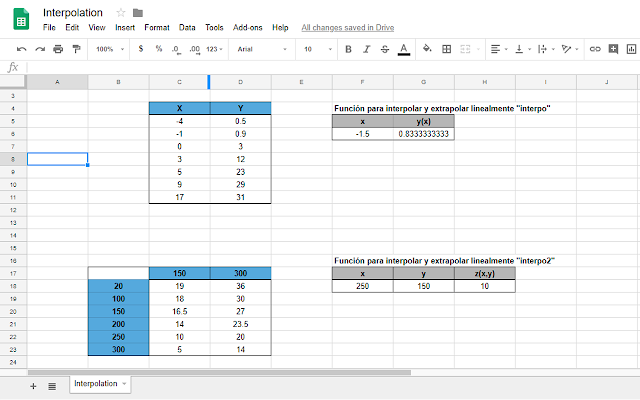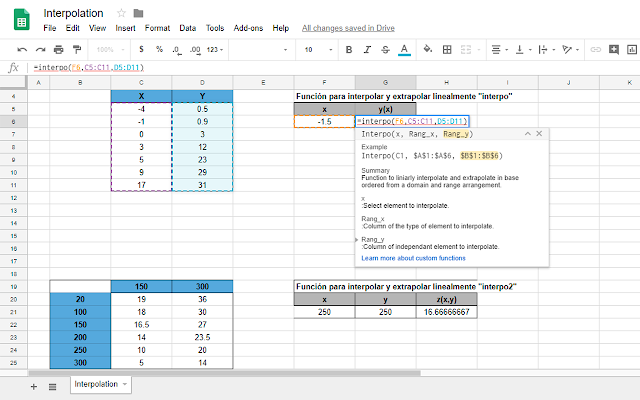Manuel Astros
•  5,059Overview
The objective of this add-in is to create easy-call functions to interpolate and extrapolate
```This add-on contain easy-call functions to interpolate and extrapolate.

By: Manuel Astros

All functions are developed to behave in a similar way that the interpolation add-in created by Jose Ivan Martinez Garcia for Excel.

It will make possible a kind of interoperability among LibreOffice-Calc, Google-sheet in interpolation matter.

Packaged Functions included in this addin until now:

interpo(x, XRange, YRange)          Linear interpolation with one parameter and two source ranges
interpo2(x, y, Range)               Linear interpolation with two parameters and a table source
cercha(x, Range, keyArg, v1, v2)    Spline interpolation with one parameter and a table source range
cerchapi(Range, keyArg, v1, v2)     Determine the initial slope (1st derivative) at the first point of first spline - Matlab ® (function csape)
cerchapf(Range, keyArg, v1, v2)     Determine the final slope at the last point of the last spline
cerchaci(Range, keyArg, v1, v2)     Determine the initial curvature (2nd derivative) at the first point of the first spline
cerchacf(Range, keyArg, v1, v2)     Determine the final curvature (2nd derivative) at the last point of the last spline
cerchara(Range, keyArg, v1, v2)     Determine the curvature radius of the segments in the given points
cercharaxy(Range, keyArg, v1, v2)   Determine the centers of curvature coordinates of the segment, in the given points
cerchap(Range, keyArg, v1, v2)      Determine the slopes (1st derivative) at the given (well-known) points.
cerchac(Range, keyArg, v1, v2)      Determine the polynomial (spline) coefficient
cerchacoef(Range, keyArg, v1, v2)   Determine the polynomial (spline) coefficient respect to the origin of coordinates
cercharea(Range, keyArg, v1, v2, w1, w2)    Determine the area under splines until the Xs axis.
cerchamx(Range, keyArg, v1, v2, w1, w2)     Determine the static moment under splines area respect the Xs axis.
cerchamy(Range, keyArg, v1, v2, w1, w2)     Determine the static moment under splines area respect the Ys axis.
cercham2x(Range, keyArg, v1, v2, w1, w2)    Determine the second static inertial moment under splines area respect the Xs axis.
cercham2y(Range, keyArg, v1, v2, w1, w2)    Determine the second static inertial moment under splines area respect the Ys axis.
cerchap2(Range, keyArg, v1, v2, w1, w2)     Determine the inertial product under the spline with respect to the Xs and Ys axes.
cerchaxg(Range, keyArg, v1, v2, w1, w2)     Determine the longitudinal coordinate of the gravity center of the area formed under the spline.
cerchayg(Range, keyArg, v1, v2, w1, w2)     Determine the vertical coordinate of the gravity center of the area formed under the spline.

Still promise a video tutorial
Complementary function of the original add-in
New functions such as linear, spline or staircase from the TSA Gnumeric plugin trying to help with interoperability with numeric```
Reviews
Language:
Sort by: